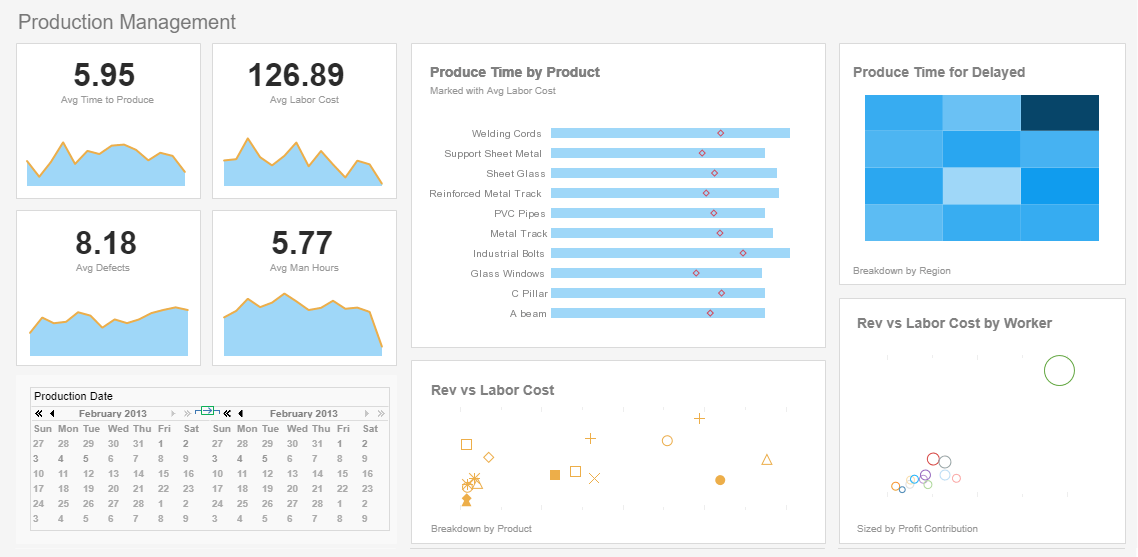# InetSoft Product Information: Graph API

This section of API documentation discusses how to control chart dimensions when embedding a dashboard into another cloud application.#1 Ranking: Read how InetSoft was rated #1 for user adoption in G2's user survey-based index

Parallel coordinates display multiple dimensions as parallel axes, rather than orthogonal axes, as for rectangular coordinates. Parallel coordinates are defined by the ParallelCoord object, which accepts a set of Scale objects as input.

To understand parallel coordinates, consider the following data set, which contains scores for three students over three consecutive tests.

 Test 1 Test 2 Test 3 Name 200 175 50 Joe 800 1000 300 Jane 10 15 20 Fred

By plotting this data on three parallel coordinates ('Test 1', 'Test 2', 'Test 3'), you can visualize trends across the different tests. To create this chart, follow the steps below:

1. Define the data set and chart objects.
``` importPackage(inetsoft.graph)
importPackage(inetsoft.graph.element)
importPackage(inetsoft.graph.scale)
importPackage(inetsoft.graph.aesthetic)
importPackage(inetsoft.graph.coord)
importPackage(inetsoft.graph.data)

var arr = [["Test1","Test2","Test3","Name"],
[100,80,20,'Joe'],[75,50,40,'Jane'],
[50,30,80,'Fred']];
dataset = new DefaultDataSet(arr);
graph = new EGraph();```
1. Create a new LineElement object to define a line-style chart, and assign its dimensions.
``` var elem = new LineElement();
1. Define the scales used for the three axes. (In this case the scales are the same for all three.)
``` var scale1 = new LinearScale("Test1");
var scale2 = new LinearScale("Test2");
var scale3 = new LinearScale("Test3");
scale1.setMax(100);
scale2.setMax(100);
scale3.setMax(100);
scale1.setMin(0);
scale2.setMin(0);
scale3.setMin(0);```
1. Create the new ParallelCoord object using the defined scales.
` var coord = new ParallelCoord(scale1,scale2,scale3);`
1. Use a CategoricalColorFrame to distinguish the three students. Assign the frame to the line element.
``` var frame = new CategoricalColorFrame("Name");
elem.setColorFrame(frame);```
1. Assign the parallel coordinate system to the chart.
``` graph.addElement(elem);
graph.setCoordinate(coord);```

The complete script is shown below:

``` importPackage(inetsoft.graph)
importPackage(inetsoft.graph.element)
importPackage(inetsoft.graph.scale)
importPackage(inetsoft.graph.aesthetic)
importPackage(inetsoft.graph.coord)
importPackage(inetsoft.graph.data)

var arr = [["Test1","Test2","Test3","Name"],
[100,80,20,'Joe'],[75,50,40,'Jane'],
[50,30,80,'Fred']];
dataset = new DefaultDataSet(arr);
graph = new EGraph();
var elem = new LineElement();
var scale1 = new LinearScale("Test1");
var scale2 = new LinearScale("Test2");
var scale3 = new LinearScale("Test3");
scale1.setMax(100);
scale2.setMax(100);
scale3.setMax(100);
scale1.setMin(0);
scale2.setMin(0);
scale3.setMin(0);
var coord = new ParallelCoord(scale1,scale2,scale3);
var frame = new CategoricalColorFrame("Name");
elem.setColorFrame(frame);Learn about the top 10 features of embedded business intelligence.